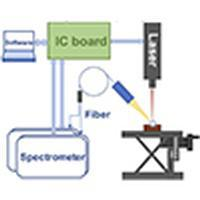Optics Express ( IF 3.561 ) Pub Date : 2020-01-15 , DOI: 10.1364/oe.381582
Haochen Li, Meizhen Huang, and Huidi XuThere are many challenges in the determination of elements in complex matrix such as soil, coal and minerals by laser induced breakdown spectroscopy (LIBS) method. Due to the influence of matrix effect, instability of laser plasma and fluctuation of laser parameters, the repeatability and accuracy of quantitative results are always not satisfactory. In order to improve the accuracy, high-energy laser (30mJ-100mJ) with precise control was utilized in many laboratories. In this paper, quantitative analysis of copper in copper concentrate by low-energy (10µJ) LIBS is studied. In order to reduce the influence of matrix effect and other factors, a partial least square regression method based on double genetic algorithm (DGA-PLS) is proposed. The detail operations are as follow: the reference spectral lines are automatically selected by GA as the optimal internal standard for spectral normalization. Then the GA is used to select variables from the normalized spectra for PLS. The results showed that, for univariate model, the coefficient of determination (R2) was improved from 0.6 to 0.97 by the optimal internal standard normalization. Compared with tradition PLS, the root mean square error of cross validation (RMSECV) and root mean square error of prediction (RMSEP) of PLS trained by the normalized spectral data decreased from 1.4% and 0.42% to 0.9% and 0.29% respectively. Compared with the normalized PLS, the RMSECV and RMSEP of the DGA-PLS trained by the normalized and feature selected spectral data decreased from 0.9% and 0.29% to 0.26% and 0.21% respectively. The results show that DGA-PLS can significantly reduce matrix effect, improve prediction accuracy and reduce the risk of overfitting in determination of copper in copper concentrate.

down
wechat
bug